# Manual segmentation in ImageJ/FIJI

## 1 Load the image sequence in FIJI/ImageJ

• Select File \rightarrowimport$\rightarrow$Image Sequence...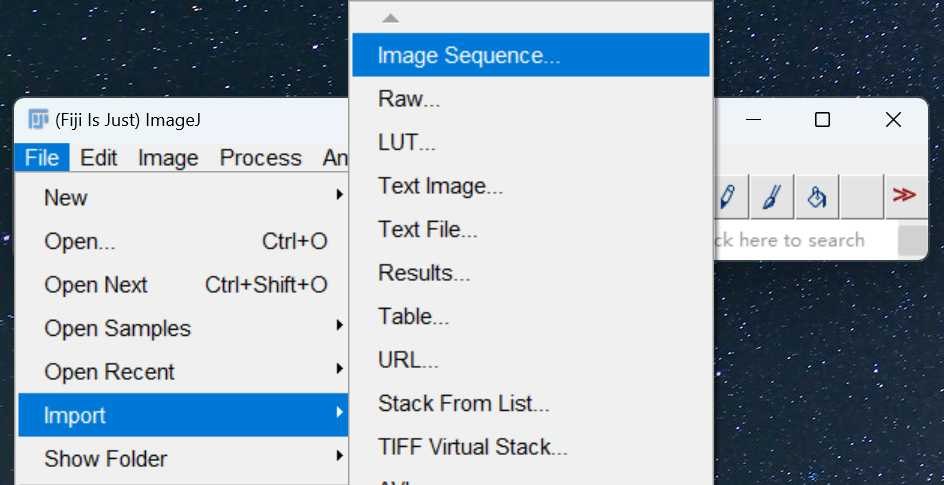• Find the directory where the image sequence is using Browse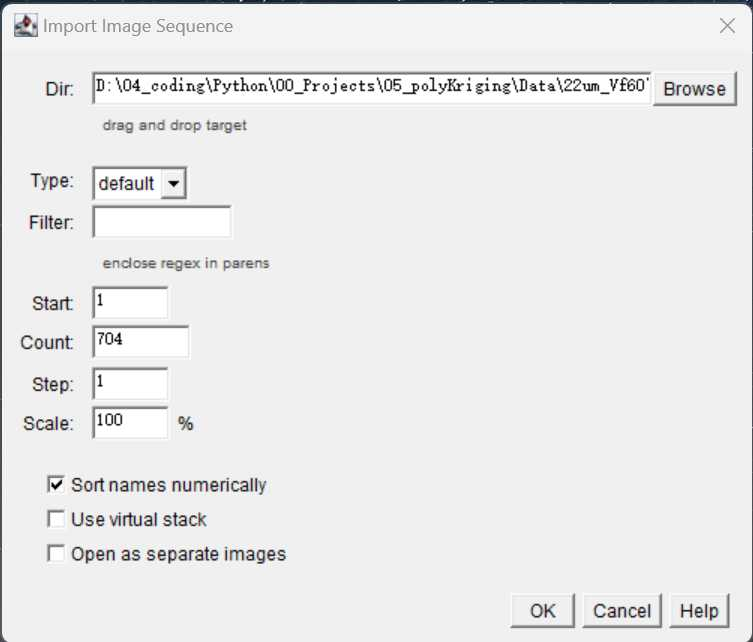• Click OK to load the image sequence

• 3D viewer for image sequence

​ Select Plugins \rightarrow3D viewer \rightarrowOK. Wait for a while you will see the 3D view of the image sequence as below: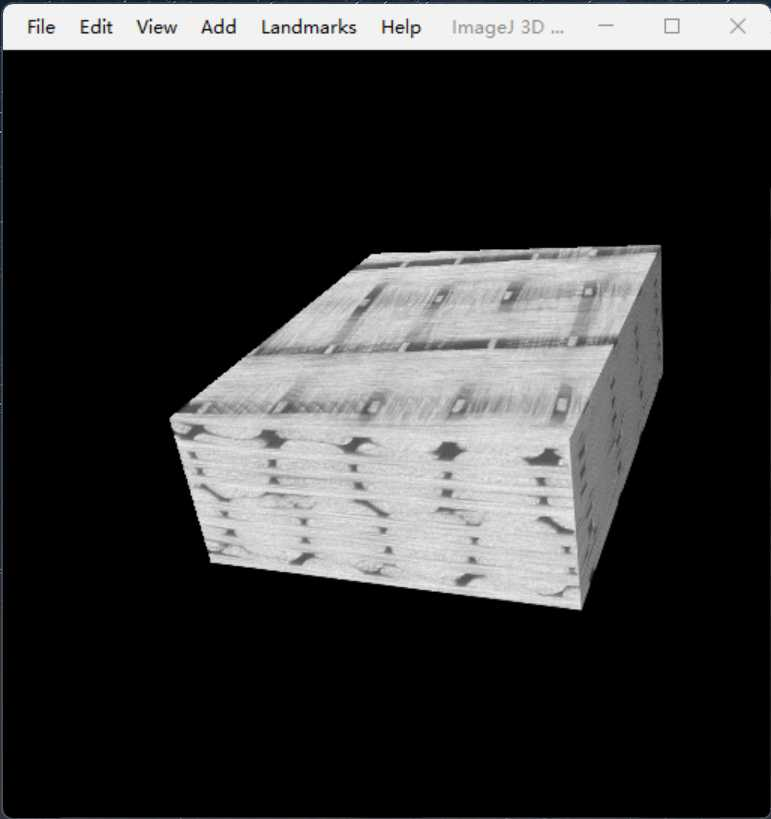## 2 Image transformation between warp and weft direction

• load the image sequence oriented in the weft direction into ImageJ;• Reslice the images as below to show the warp tows and binders;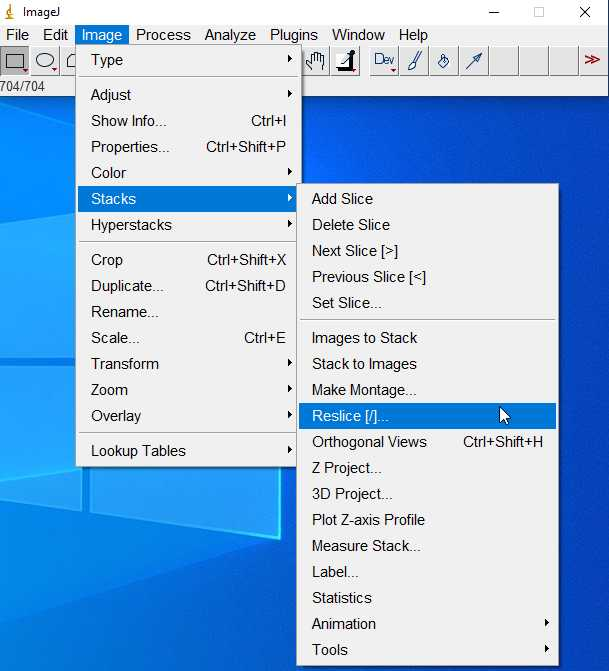You should get:• Recover to the original weft view: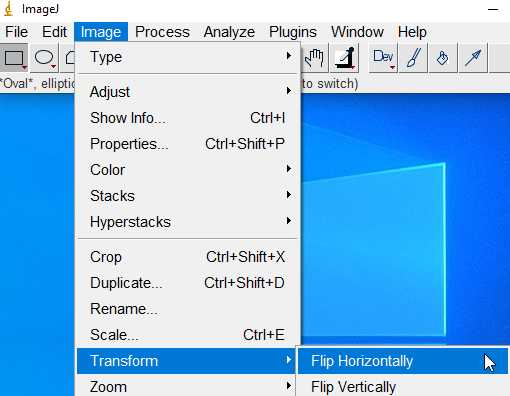## 3 Manual selection of ROI

ROI: Region of Interest

• A label is assigned to each fiber tow. In the case of warp tows, for instance, the labels are assigned as below:Let's use Tow 8 to show the procedure of manual segmentation.

## 4 Import existing selections:

1. Load the image sequence which the ROI information belonging to.
2. Drag and put the ROI file (.zip file containing multi .roi files)

If the ROI information was stored in csv files, the following code to can be used in imageJ (save the code as xxx.ijm file) to import.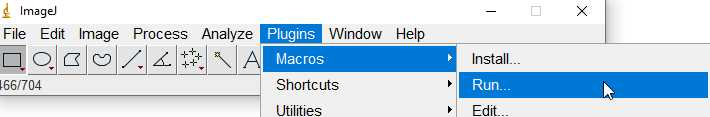### csv to ROI

run("Select None");//Remove all the selections
roiManager("reset");//Reset the ROI manager
dir_ROI = getDirectory("Choose a Directory to import")
list = getFileList(dir_ROI);
image_roi = getTitle();

for(i = 0; i < list.length; i++){

Table.open(dir_ROI+'Long_0_F_'+ 4*i+1 +'.csv');
xpoints = Table.getColumn("x");
ypoints = Table.getColumn("y");

zpoints = Table.get("z", 2);
print(zpoints);

selectWindow(image_roi);
setSlice(zpoints);
makeSelection("polygon", xpoints, ypoints);
roi_name = "0"+zpoints;
Roi.setName(roi_name);
roiManager("select", i)
RoiManager.setPosition(zpoints);
close(Table.title);
}


## 5 Export the selections (ROI) to csv and .npz fileThen run the following code that stored in a xxxx.ijm file:

### 5.1 ROI to csv

ROI_output_csv.ijm code for imageJ

//index for tow index/label
dir_saving = getDirectory("Choose a Directory to save");

for(index = 8; index < 32; index++){

dir_saving2 = dir_saving + "weft_" + index +"/";

roiManager("Open", "C:/Users/AQ84510/Nutstore/2/04_coding (2)/Python/00_Projects/05_polyKriging/Data/22um_Vf60/04_raw_data_pcd/weft/roi/weft_" + index + ".zip");

run("Clear Results");
n = roiManager('count');
for (i = 0; i < n; i++) {
run("Clear Results");
roiManager('select', i);
getSelectionCoordinates(x, y);
z=getSliceNumber();
for (j=0; j<x.length; j++) {
setResult("x", j, x[j]);
setResult("y", j, y[j]);
setResult("z", j, z);
}
setOption("ShowRowNumbers", true);
updateResults;
z=z-1; // The filenames start from 0 to be consistent with Python
saveAs("Results", dir_saving2 +'weft_'+index + '_'+z+'.csv');}

roiManager("Deselect");
roiManager("Delete");
}


A single csv file contains the key points/pixel positions to describe a cross-section of a fiber tow in the following format: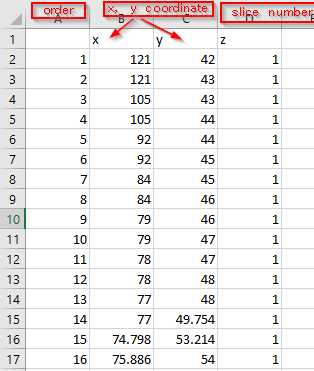### 5.2 csv to pcd

csv2pcd_DataPreparation.py

.pcd is a new defined format for point cloud dataset.

from polykriging import utility
import polykriging as pk
import numpy as np
import zipfile, os
import pandas as pd

# 压缩某个目录下所有文件
def compress_file(zipfilename, dirname):
# zipfilename是压缩包名字，dirname是要打包的目录
if os.path.isfile(dirname):
with zipfile.ZipFile(zipfilename, 'w') as z:
z.write(dirname)
else:
with zipfile.ZipFile(zipfilename, 'w') as z:
for root, dirs, files in os.walk(dirname):
for single_file in files:
if single_file != zipfilename:
filepath = os.path.join(root, single_file)
z.write(filepath)

path = utility.choose_directory(titl =
"Choose the directory that contains ROIs stored in csv files")
cwd = utility.cwd_chdir(path)
files = utility.filenames(path)

yarn = files[:files.rfind("_")]

compress_file(yarn + ".zip", "./")
for i in np.arange(len(files)):
section = files[ : files.rfind("_") + 1] + str(i+1) + ".csv"